# E x c e l

CPT 105 · Franklin College · Erich Prisner · 2002-2007## Contents:

### 1. Worksheets, Rows, Columns, Cells, Addresses

Excel is a spreadsheet program. An Excel file is called workbook. It contains one or several pages, called worksheets. Each worksheet is divided into columns and rows. The small rectangles (intersection of rows and columns) are the cells. Columns are labeled by "A", "B", "C", ... rows by "1", "2", 3", ... You can read off the address of the cell by its coordinates, as A4, B4, and so on.

### 2. Cells

Cells have a certain content---what is showing---and a certain formatting---how it is displayed. Note that the content of a cell is different from its address.Into cells you can type one of the following:

• either a number. Then the value of the cell is the number. Numbers could also be percentages, (like those in columns E to J in the example above) or dates (like those in columns C and D in the example above), ... if you format the cell right. These cells are also called data or input cells. Their value may change, the the user will replace their value by the updated value.
• or text, a so-called label. Then the cell doesn't have a value.
• or a formula/function. Formulas always start with the equal sign "=". These cells are called output cells. Their value depends on the value of the input cells. If the input changes, Excel will immediately and automatically recompute the displayed value of the output cells. That is the essence of Excel.

Example: Download the Excel file, save it into your "My Documents" folder and open it from there (see also the picture above). It is a simple sheet displaying how your money grows on the bank.The yellow cells are the main input cells (but B5, B6, ... B19 are input cells too), the orange ones are the output cells. Type something deifferent in the yellow cells (choose a different interest rate and a different principal) and see how the displayed values of the output cells change automatically.

## What you should be able to do

###Please check the menus and submenus. As usual with Office programs, you can copy and paste contents of cells using "Ctrl-c" or the copy button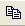and "Ctrl-v" or the paste button. Use also the cut buttonor "Ctrl-x". You can also copy whole blocks. Note that the widths and heights (in number of cells) of the blocks must coincide. When you copy, both contents and formatting are copied. (If you want to avoid this, try the menu "Edit|Paste Special" and check what you want to paste.)

Very important is the Edit|Undo menu or Undo button. Use it!

### 4. Selecting cells, navigating,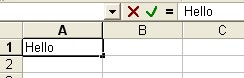Click on any cell. A bold border around the cell shows, to indicate that the cell is selcted. Now type something. The text or number is shown in the cell and in the formula bar (see the upper "Hello" in the example. The cancel and enter buttonsare visible when in edit mode. You leave the cell by pressing the "enter" button, or by pressing the "enter" key.

You can navigate by clicking with the mouse somewhere else, or by using the arrow keys.

If you want to edit text or numbers in cells, you have to select it again, and then go with the mouse in the formular bar to get a cursor. No cursor will show if you move the mouse over the selected cell.

You select a whole row by clicking on its row number (in the gray border area). Likewise columns are selected by clicking on the column letter in the grey area.

### 5. Data Types:

Let's assume you type the number 0.654321 into a cell. Depending on the formatting of the cell, it may displayed as 0.654321 or 0.65 or 65% or 0.65\$.

• Numbers: If the cell is formatted as a number, you can choose how many digits after the decimal point are displayed.
• Percentages. Note that 65% means 0.65. Again you can choose the number of digits.
• Currencies. Whenever numbers mean money, it is appropriate to include the currency symbol (\$, SFr, ...) into the cell. Also, if you work with currency, you don't want to have entries like \$20.3 or \$20.354.
• Dates: are treated like numbers. A date is the number of days from January 1, 1900 (n Excel for Macintosh, it's 1904!) until the corresponding date. Date and Time is stored internally as a real number.

### 6. Formatting Cells:

To format cells, you should use one of the following:

• The Formatting Toolbar: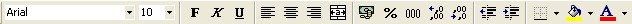• The Format Cells dialog box: Choose "Format|Cell" in the menus. A dialog box with 6 tabs will pop up. In the first one, "number" you choose the data type. In the second one, Alignment, you select the alignment, word wrapping, you can also merge several cells there. Then there are tabs for font, border, pattern (background) and protection.
• The Format Painter works like in Word. Click on the cell whose formatting you want to copy, click on the Format Painter button, and paint over those cells which you want to format.
• (Styles)

### 7. Formatting the Whole File

• You can change column width or row height by using the Format|Row or Format|Column menu, or by grabbing and dragging the lines between the column letters or between the row numbers in the grey area. Doubleclicking on such a line will collapse the column width or row height to the needed space.
• To insert a row, select the row above which you want to insert. Choose the menu "Insert|Row". Inserting columns works similar.
• freezing,
• You can also insert a new worksheet.
• deleting cells
• merging cells

### 8. Conditional Formatting

The format of the cell changes, depending either on the value of the cell itself ("cell value is"), or depending on the value of other cells ("formula is"). Click the menu "Format|Conditional Formatting". Fill out the form, and the click "Format" to choose the format you want in case the condition is fulfilled.

### 9. Formulas

Formulas or function almost always depend on the values of other cells. These other cells are referred to by their address. With formulas, the values of some cells are used to compute the value of the present cell (in which the formula is written). Note that the formula is written in this cell, but what is displayed is the value of the cell, the result of this formula. Formulas always start with an "="-sign.

Formulas should almost always contain the addresses instead of the data (numbers)! The reason is that these input data numbers may change. The output values will only change automatically if the formula refers to the corresponding cell by its address. Type cells address into formulas by clicking on the corresponding cell or by typing in the cell address.

### 10. Basic Mathematical operations:

For addition, subtraction, multiplication, division, simply use +, -, *, /. Don't forget to use parantheses. The formulas "=A2+A3*A4" and "=(A2+A3)*A4" have different results. Since dates are (internally) numbers, you can also add, subtract, multiply, or divide them.

In the "Money on the Bank" example above, the formula in cell D5 is "=D3*(1+D2)", and the formula in cell D6 is "=D5*(1+D2)".

### 11. Copying Formulas or Functions:

If you copy a formula to some other location, the formula changes. Let's assume you have numbers in the cells A1, A2, A3, B1, B2, B3, and the formula "=A1+B1" in cell C1. Then the displayed value in cell C1 is the sume of the numbers in A1 and B1. If you copy the formula in cell C1 into cell C2, the formula changes into "=A2+B2". Excel makes kind of intelligent changes here, it assumes that C1 relates to A1 and B1, just as C2 relates to A2 and B2. In other words: Excel computes the relative position of each reference, and tries to keep these relative positions.

You can also copy one formula to a whole block. Another fast way of copying numbers or formulas to a column or row is the fill down and right feature. You select the cell which you want to copy. Then you grab the small black square in the lower right corner, the fill handle, and drag it down or to the right,

• relative referencing (addressing): Formulas refer to cells, like D14, or to cell ranges, like D14:D22, for instance. If this formula is copied one row down, it changes automatically into D15, respectively D15:D23. If you copy the formula one the right, you get reference to E14 respectively E14:E22. This is relative referencing---Excel just remembers the relative distance from the actual cell (where the formula is in) to D14 like "two up, three to the left", and keeps this rule in all copied formulas.
• absolute referencing (addressing): If you don't want Excel to change either row number, or column letter, or both, in your formulas, write a \$-sign in front of it, like in E\$14, or \$E14, or \$E\$14.

In the "Money on the Bank" example above, if you write the formula "=D5*(1+D2)" into cell D6 and copy it down to D7, D8, ... the formulas change into "=D6*(1+D3)", "=D7*(1+D4)", ... which don't make sense at all. Changing the address D5 to D6 and D7 makes sense, here relative referencing is appropriate. But the interest rate is always in D2, so this address should never change. Therefore, we rather type the formula "=D5*(1+\$D\$2)" into cell D6. When copying this version down to cells D7, D8, ... we obtain the correct formulas "=D6*(1+\$D\$2)", "=D7*(1+\$D\$2)", ....

### 12. Functions as prepackaged formulas.

Functions are abbreviations for (more or less complicated) formulas. For instance, instead of typing in "=A1+A2+A3+A4", you could use the function "=SUM(A1:A4)". Instead of the formula "=(A1+A2+A3+A4)/4", you could use the formula "=AVERAGE(A1:A4)". Functions consist of function name (like "SUM" and arguments in parantheses. You can type the function, or use the function wizard to create the text, in case you don't remember the function name or the syntax.

• A range is a rectangular block of cells. You highlight such such a block either by clicking and dragging or by clicking, keeping the shift key pressed, and clicking in the other corner of the rectangle. Many functions have some range as argument. Ranges are abbreviated by address-of-left-upper-corner:address-of-right-lower-corner, like A1:F4.
• To create a function with the function wizard, you select the cell where you want to write the function in, and then you press this button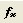on the general toolbar. Functions are grouped under certain categories. Brief descriptions are given, and the wizard also prompts you to type in the argument ranges. Instead of typing these ranges, you may want to select the corresponding range with your mouse when prompted.

In the "Money on the Bank" example above, we need the exponential function EXP() for F5, F6, ... We type "=\$D\$3*EXP(\$D\$2*B5)" into cell F5 and copy it down to F6, F7, ... to get "=\$D\$3*EXP(\$D\$2*B6)" and "=\$D\$3*EXP(\$D\$2*B7)" there. For refering to interest rate and principal we use absolute referencing, but to time (B5) we refer relatively.

### 13. Important Functions

• SUM has a special shortcut button: It has the form "=SUM(range)", where "range" is the range of cells whose values should be added.
• AVERAGE: It has the form "=AVERAGE(range)".
• IF: It has the form "=IF(condition,valueiftrue,valueiffalse)". condition is a logical condition, like "A1>0", for instance. valueiftrue and valueiffalse are numbers or formulas. The number or value of valueiftrue is displayed if the condition is true, and otherwise the number valueiffalse is displayed.

### 13b. More Functions

• MAX has the form "=MAX(range)" and displays the largest number of the range.
• COUNT has the form "=COUNT(range)" and displays the number of numbers in the range.
• PMT

### 14. Sorting

You can sort the rows of some table, depending on the values in some column. Just highlight the whole table, and choose the menu Data|Sort. Decide based on which column you want to sort, and do it. If it's just one column, you can also use these buttons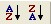on the standard toolbar.

### 15. Charts

Highlight a table, preferably including labels, and click on the Chart Wizard button. Select Bar, Line, or Pie Chart, and select whether you want to view the rows or the columns as independent variable.

To insert a data series (row or column, depending on your chart) to your existing chart, you select (highlight) the data celss to include, copy the whole part, click on the chart and paste.

To insert labels on the x-axis, you select the row or column containing the labels, copy it, click on the x-axis on the existing chart, and paste.

## Some more features

### Statistics

Go to Tools|Data Analysis and choose.

### Several Worksheets

Each file contains several pages (sheet1, sheet2, ... visible in the left bottom corner. Click on that, on you turn pages to some new sheet. You may also rename the sheets, shuffle them, and you may, of course, reference between sheets.

### Filter

are similar to queries in databases.